Engineering Jobs   »   Electrical Engineering quizs   »   GATE 2022. GATE ELECTRICAL,

# GATE’22 EE: Daily Practices Quiz 20-Sep-2021

## GATE 2022 Important Dates

Organizing Institute for GATE 2022 is the Indian Institute of Technology Kharagpur. For GATE 2022 Exam IIT Kharagpur has released the official notification and Important dates regarding the GATE 2022 Exam. Aspirants preparing for GATE 2022 must check the details regarding GATE 2022 Important Dates.

## GATE’22 EE: Daily Practices Quiz

GATE’22 EE: Daily Practices Quiz 20-Sep-2021

Each question carries 2 marks
Negative marking: 2/3 mark

Total Questions: 05
Total marks: 10
Time: 12 min.

Q1. An overhead transmission lines the effect of capacitance can be neglected when the length of line is less than
(a) 200 km
(b) 160 km
(c) 100 km
(d) 80 km

Q2. A 3- ϕtransmission line uses 1.24 cm diameter conductor and these are placed at corners as shown below of 2 m. The inductance/phase is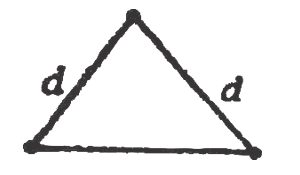(a) 12×10^(-7) H
(b) 8.4 × 10^(-7) H
(c) 7.6 × 10^(-6) H
(d) 12.5 × 10^(-6) H

Q3. The rotor bar current in a three-phase squirrel cage induction motor is 100 Amp. The rotor resistance is 0.05 Ohm/phase. At a slip of 5% the air gap power is
(a) 5 kW
(b) 10 kW
(c) 30 kW
(d) 60 kW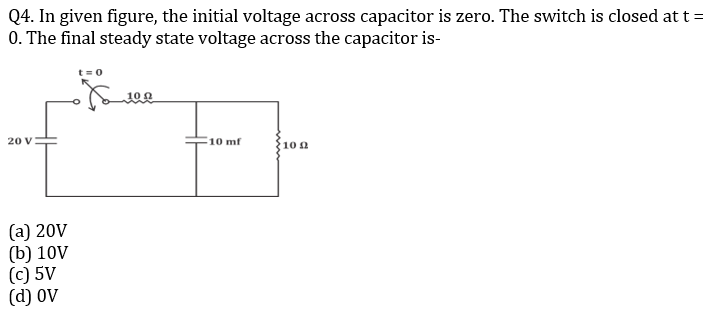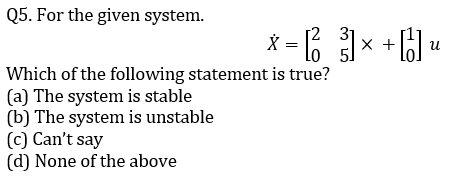## SOLUTIONS

S1. Ans.(d)

Sol.

 Type of Transmission If f = 50 Hz then, length is Effect of capacitance Short line L < 80 km Neglected Medium line 80 km < L < 240 km The capacitor is lumped and constant Long line L > 240 km Capacitance is uniformly distributed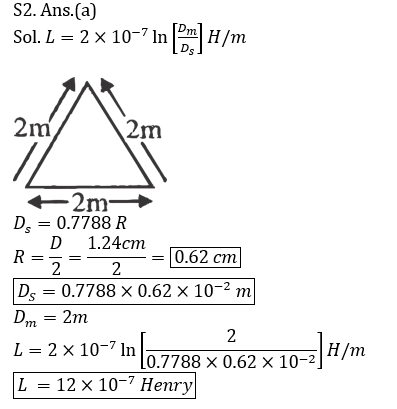S3. Ans.(c)
Sol. Given, Rotor current (I_2 ) = 100 A
Rotor resistance (R_2 ) = 0.05 Ω\/Phase
Slip = 5 % = 0.05 pu
Air gap power (Pg.) =?
Copper Loss (P_cu ) = 3 (I_2 )^2 R_2
= 3 × (100) ² × 0.05
= 3 × 10^4 × 0.05
= 1500 W
Air gap power of 3 – ϕ squirrel cage induction motor is given by-
P_g=P_cu/S=1500/0.05
= 3 × 10^4
= 30 kW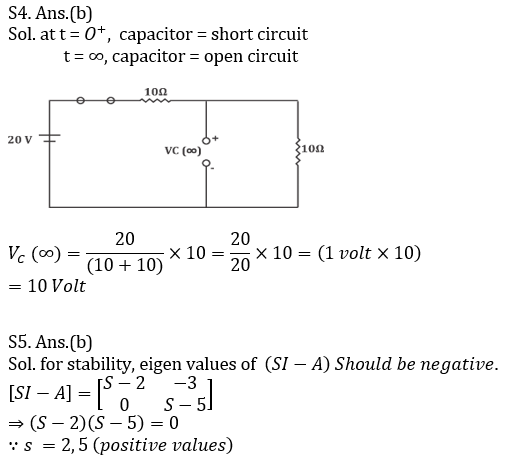Sharing is caring!

Thank You, Your details have been submitted we will get back to you.